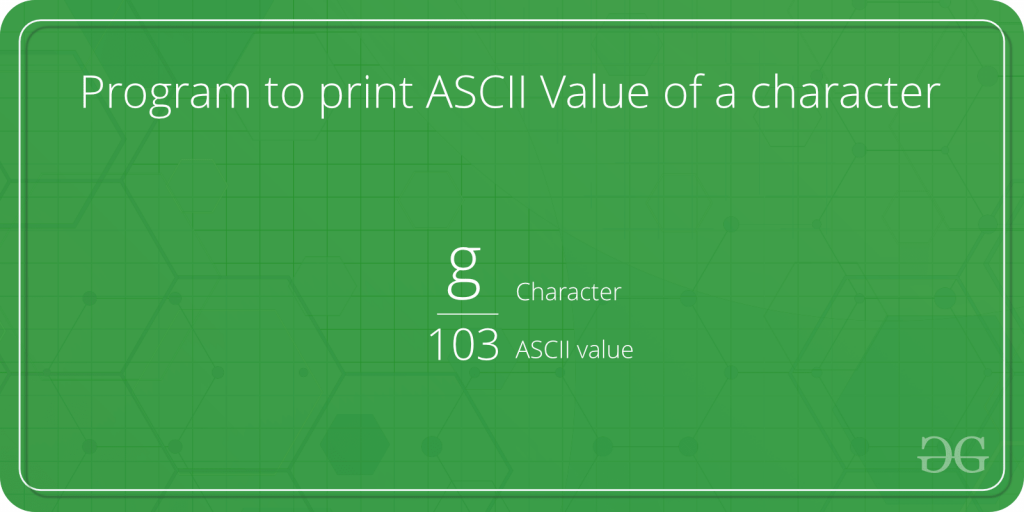# Program to print ASCII Value of a character

• Difficulty Level : Easy
• Last Updated : 19 Aug, 2021

Given a character, we need to print its ASCII value in C/C++/Java/Python.Attention reader! All those who say programming isn't for kids, just haven't met the right mentors yet. Join the  Demo Class for First Step to Coding Coursespecifically designed for students of class 8 to 12.

The students will get to learn more about the world of programming in these free classes which will definitely help them in making a wise career choice in the future.

Examples :

```Input : a
Output : 97

Input : D
Output : 68```

Here are few methods in different programming languages to print the ASCII value of a given character :

1. Python code using ord function :
ord(): It converts the given string of length one, returns an integer representing the Unicode code point of the character. For example, ord(‘a’) returns the integer 97.

## Python

 `# Python program to print``# ASCII Value of Character` `# In c we can assign different``# characters of which we want ASCII value` `c ``=` `'g'``# print the ASCII value of assigned character in c``print``(``"The ASCII value of '"` `+` `c ``+` `"' is"``, ``ord``(c))`
1. Output:

`The ASCII value of g is 103`
1.
2. C code: We use format specifier here to give the numeric value of character. Here %d is used to convert character to its ASCII value.

## C

 `// C program to print``// ASCII Value of Character``#include ``int` `main()``{``    ``char` `c = ``'k'``;` `    ``// %d displays the integer value of a character``    ``// %c displays the actual character``    ``printf``(``"The ASCII value of %c is %d"``, c, c);``    ``return` `0;``}`
1. Output:

`The ASCII value of k is 107`
1.
2. C++ code: Here int() is used to convert character to its ASCII value.

## CPP

 `// CPP program to print``// ASCII Value of Character``#include ``using` `namespace` `std;``int` `main()``{``    ``char` `c = ``'A'``;``    ``cout << ``"The ASCII value of "` `<< c << ``" is "` `<< ``int``(c);``    ``return` `0;``}`
1. Output:

`The ASCII value of A is 65`
1.
2. Java code: Here, to find the ASCII value of c, we just assign c to an int variable ASCII. Internally, Java converts the character value to an ASCII value.

## Java

 `// Java program to print``// ASCII Value of Character``public` `class` `AsciiValue {` `    ``public` `static` `void` `main(String[] args)``    ``{` `        ``char` `c = ``'e'``;``        ``int` `ascii = c;``        ``System.out.println(``"The ASCII value of "` `+ c + ``" is: "` `+ ascii);``    ``}``}`
1.
2. C# code : Here, to find the ASCII value of c, we just assign c to an int variable ascii. Internally, C# converts the character value to an ASCII value.

## CSHARP

 `// C# program to print``// ASCII Value of Character``using` `System;` `public` `class` `AsciiValue``{``    ``public` `static` `void` `Main()``    ``{` `        ``char` `c = ``'e'``;``        ``int` `ascii = c;``        ``Console.Write(``"The ASCII value of "` `+``                        ``c + ``" is: "` `+ ascii);``    ``}``}` `// This code is contributed``// by nitin mittal`
1. Output:

`The ASCII value of e is 101`

Here is a method to print the ASCII value of the characters in a string using python:

## Python3

 `print``(``"Enter a String: "``, end``=``"")``text ``=` `input``()``textlength ``=` `len``(text)``for` `char ``in` `text:``    ``ascii ``=` `ord``(char)``    ``print``(char, ``"\t"``, ascii)`

Input:

`Enter a String: Aditya Trivedi`

Output:

```A      65
d      100
i      105
t      116
y      121
a      97
32
T      84
r      114
i      105
v      118
e      101
d      100
i      105```

My Personal Notes arrow_drop_up